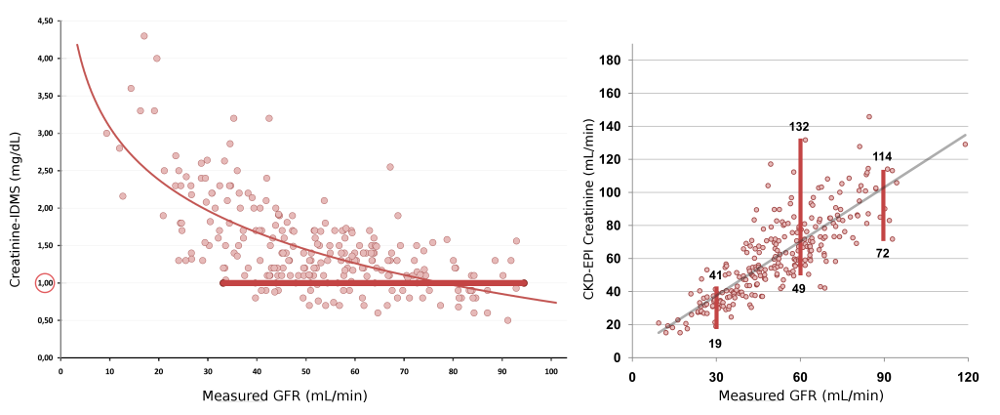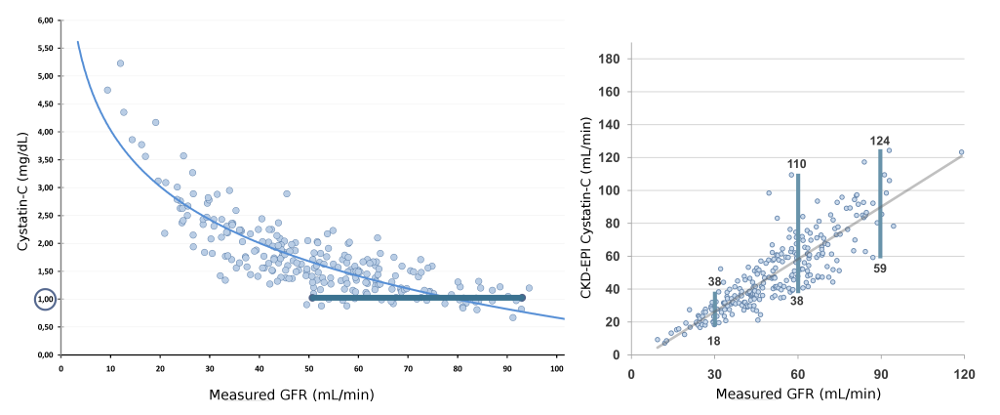### Estimation of renal function

In clinical practice, renal function is frequently estimated by indirect methods using endogenous markers such as serum creatinine, which is included in mathematical algorithms along with other variables such as age, weight , height or sex. In the last 70 years, more than 40 formulas based on creatinine have been published. However, none of them have shown acceptable accuracy and precisión in reflecting renal function. The average error is about ±30% of real renal function. This is unacceptable in certain clinical situations in which a reliable evaluation of renal function is important.(Left) Curvilinear and inverse relationship between serum creatinine and renal function measured by the plasma clearance of iohexol. For the same creatinine value (1.00 mg / dL), measured renal function can range between 35 and 95 mL/min; (Right) Relationship between the estimation of renal function by creatinine-based CKD-EPI formula and renal function measured by the plasma clearance of iohexol. For the same GFR value of 30 ml/min, estimated renal function can range between 19 and 41 mL/min. Similarly, for a GFR value of 60 ml/min, estimated renal function may range between 49 and 132 mL/min and for a GFR value of 90 mL/min, estimated renal function may range between 72 and 114 mL/min.

Since 2000 and trying to overcome the error of creatinine-based formulas, more than 20 equations have been developed based on a new renal function marker: cystatin-C. However, the error of formulas base don cystatin-C remains as large as that observed with creatinine-based equations. Thus, no real progresses has been shown in eGFR in the last 70 years.(Left) Curvilinear and inverse relationship between serum cystatin-C and renal function measured by the plasma clearance of iohexol. For the same cystatin-C value (1.00 mg/L), measured renal function can range between 50 and 95 mL/min; (Right) Relationship between the estimation of renal function by cystatin-C-based CKD-EPI formula and renal function measured by the plasma clearance of iohexol. For the same GFR value of 30 ml/min, estimated renal function can range between 18 and 38 mL/min. Similarly, for a GFR value of 60 ml/min, estimated renal function may range between 38 and 110 mL/min and for a GFR value of 90 mL/min, estimated renal function may range between 59 and 124 mL/min.